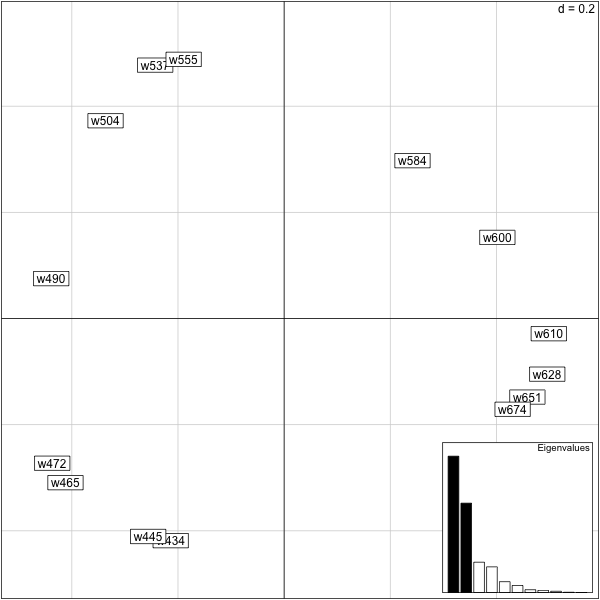# PCA, SVD and MDS¶

## SVD notation and example¶

$\begin{split}{\bf X}_{n\times p}= U_{n\times p}S_{p\times p}V_{p\times p}', V'V=I_p, U'U=I_p. {\bf S}_{p\times p}= \left(\begin{array}{lccr} s_1 &0& \cdots& 0\\ 0 & s_2 & \cdots& 0\\ 0 & \cdots & s_{k-1}& 0\\ 0 & 0 & \cdots& s_{k}\\ \end{array} \right)\end{split}$

where r is the rank of X.

### Generating a rank three matrix¶

Now we have generated an approximately rank three matrix.

>  load("/Users/susan/sphinx/data/SVD3ex.save")
>  svd(X3)$d  7.000000e+00 5.000000e+00 3.000000e+00 5.010064e-16 4.021512e-16  1.811002e-16 > res3=eigen(t(X3)%*%X3) > svd(X3)$d^2
 4.900000e+01 2.500000e+01 9.000000e+00 2.510074e-31 1.617256e-31
 3.279727e-32
>  X3.pca=dudi.pca(X3,scannf=F,nf=4)
>  X3.pca$eig  3.7555053 2.2097216 0.0347731 > X3.pca$co
Comp1       Comp2      Comp3
V1 -0.7575077  0.63614665 0.14662713
V2 -0.8207017 -0.57009027 0.03802406
V3 -0.4067514 -0.91303611 0.03030472
V4  0.6277681 -0.77564837 0.06539690
V5  0.9958962 -0.06702507 0.06081494
V6  0.9781551  0.20069475 0.05416792
>


So, the output from the SVD, Eigendecomposition and PCA are not the same?

#### Why Not?¶

Well, for PCA the default is for the matrix to be centered by columns first, so if we don’t do that, we should get the same as above, let’s try it.

> load("/Users/susan/sphinx/data/SVD3ex.save")
> X3.pca=dudi.pca(X3,scannf=F,nf=4,center=F)
> X3.pca$co Comp1 Comp2 Comp3 V1 -0.3660850 0.7285115 0.57901015 V2 -0.7381575 0.5827776 -0.33984369 V3 -0.9403123 0.1881923 -0.28354248 V4 -0.9870967 -0.1504679 -0.05476738 V5 -0.9225645 -0.3586531 0.14227646 V6 -0.8400384 -0.4695396 0.27178662 > res3$vectors[,1]
 -0.7364928 -0.4432826 -0.3274789 -0.2626469 -0.2204199 -0.1904420
>


Not right yet, so not only does the dudi.pca center the data but by default it also rescales it so that all the variables (columns) have variance 1, so let’s try not rescaling or centering.

> load("/Users/susan/sphinx/data/SVD3ex.save")
> X3.pca=dudi.pca(X3,scannf=F,nf=4,center=F,scale=F)
> X3.pca$co[,1]  1.7184832 1.0343260 0.7641174 0.6128427 0.5143130 0.4443648 > sum(X3.pca$co[,1]^2)
 5.444444
> X3.pca$eig  5.444444 2.777778 1.000000 >  and see that by coincidence the first eigenvalue matches the norm of the component in the co (columns) part of X3.pca. In fact if we look at the normalized component which is in X3.pca$c1, we have an exact match:

> load("/Users/susan/sphinx/data/SVD3ex.save")
> X3.pca=dudi.pca(X3,scannf=F,nf=4,center=F,scale=F)
> X3.pca$c1 CS1 CS2 CS3 V1 0.7364928 0.6225002 -0.2550021 V2 0.4432826 -0.1818705 0.6866860 V3 0.3274789 -0.3508553 0.2611139 V4 0.2626469 -0.3921783 -0.1043599 V5 0.2204199 -0.3945644 -0.3509658 V6 0.1904420 -0.3831871 -0.5110654 > res3$vectors[,1]
 -0.7364928 -0.4432826 -0.3274789 -0.2626469 -0.2204199 -0.1904420
>


Now we can make the eigendecomposition match in the other direction: Start with X3 then center and scale it and check that its svd and eigenvectors correspond to the simple PCA:

> load("/Users/susan/sphinx/data/SVD3ex.save")
> X3sc=scale(X3)
> apply(X3sc,2,mean)
 -2.464151e-17  5.551115e-17 -2.463548e-17  1.853534e-17 -1.048424e-16
  7.250903e-17
> apply(X3sc,2,sd)
 1 1 1 1 1 1
> eigen(t(X3sc)%*%X3sc)
$values  3.004404e+01 1.767777e+01 2.781848e-01 1.036812e-15 4.897449e-16  -8.461922e-16$vectors
[,1]        [,2]      [,3]       [,4]        [,5]       [,6]
[1,] -0.3908885  0.42794551 0.7863079  0.0000000  0.00000000  0.2139829
[2,] -0.4234978 -0.38350838 0.2039092  0.2289084 -0.09038418 -0.7559257
[3,] -0.2098915 -0.61421324 0.1625132 -0.6840457  0.15154090  0.2477779
[4,]  0.3239404 -0.52179043 0.3506998  0.6044730  0.11955955  0.3465904
[5,]  0.5139015 -0.04508878 0.3261284 -0.2623778 -0.72797696 -0.1694697
[6,]  0.5047468  0.13501040 0.2904828 -0.2131961  0.65162958 -0.4153901

> res3c=dudi.pca(X3sc,nf=6,scannf=F)
> res3c$c1 CS1 CS2 CS3 V1 -0.3908885 0.42794551 0.7863079 V2 -0.4234978 -0.38350838 0.2039092 V3 -0.2098915 -0.61421324 0.1625132 V4 0.3239404 -0.52179043 0.3506998 V5 0.5139015 -0.04508878 0.3261284 V6 0.5047468 0.13501040 0.2904828 > res3c$eig
 3.7555053 2.2097216 0.0347731
> eigen(t(X3sc)%*%X3sc)$values/res3c$eig
 8
>


### Summary of things we noticed¶

1. Usually PCA does an eigendecomposition of the crossproduct of the standardized, recentered matrix divided by (n-1), we call this matrix the correlation matrix.
2. The square of the singular values are the eigenvalues.
3. The principal components have their norm equal to the eigenvalue.
4. Each eigenvalue actually gives the variance explained by that component (ie. the first eigenvalue gives the variance explained by the first component)

### R code for cut and paste¶

rm(X)
svd(X3)$d res3=eigen(t(X3)%*%X3) svd(X3)$d^2
#Comparing   the ouput from a PCA and an SVD?
X3.pca=dudi.pca(X3,scannf=F,nf=4)
X3.pca$eig X3.pca$co
X3.pca=dudi.pca(X3,scannf=F,nf=4,center=F)
X3.pca$co res3$vectors[,1]
X3.pca=dudi.pca(X3,scannf=F,nf=4,center=F,scale=F)
X3.pca$co[,1] ##still not right, but we can remark a funny thing, ##the sums of squares of this vector are equal to: sum(X3.pca$co[,1]^2)
####we compare this to
X3.pca$eig load("/Users/susan/sphinx/data/SVD3ex.save") require(ade4) X3.pca=dudi.pca(X3,scannf=F,nf=4,center=F,scale=F) X3.pca$c1
res3$vectors[,1] load("/Users/susan/sphinx/data/SVD3ex.save") require(ade4) X3sc=scale(X3) apply(X3sc,2,mean) apply(X3sc,2,sd) eigen(t(X3sc)%*%X3sc) res3c=dudi.pca(X3sc,nf=6,scannf=F) res3c$c1
res3c$eig ##What's the relationship between the eigenvalues, ###there must be one (the evectors are the same) eigen(t(X3sc)%*%X3sc)$values/res3c$eig  ## PCA and MDS(multidimensional scaling)¶ We want to compute a matrix of distances between rows of X: > load("/Users/susan/sphinx/data/SVD3ex.save") > X3c=sweep(X3,2,apply(X3,2,mean)) > B=crossprod(t(X3c)) > DX2=as.matrix(dist(X3c))^2 > DX2c=sweep(DX2,1,apply(DX2,1,mean)) > cDX2c=sweep(DX2c,2,apply(DX2c,2,mean)) > apply(cDX2c,1,mean) 1 2 3 4 5 1.185202e-15 -3.455955e-16 -4.933361e-16 1.974031e-16 -6.413176e-16 6 7 8 9 -6.910464e-16 -7.896365e-16 -2.961077e-16 0.000000e+00 > apply(cDX2c,2,mean) 1 2 3 4 5 -1.579369e-15 0.000000e+00 -1.481020e-16 0.000000e+00 -1.972284e-16 6 7 8 9 0.000000e+00 -1.477888e-16 -4.943962e-17 2.470053e-16 > B[1:4,1:4] [,1] [,2] [,3] [,4] [1,] 25.982036 2.700244 -2.2400063 -3.8056073 [2,] 2.700244 5.585367 2.6459863 0.4513750 [3,] -2.240006 2.645986 1.7776930 0.8125225 [4,] -3.805607 0.451375 0.8125225 0.7202679 > cDX2c[1:4,1:4] 1 2 3 4 1 -51.964071 -5.400488 4.480013 7.611215 2 -5.400488 -11.170735 -5.291973 -0.902750 3 4.480013 -5.291973 -3.555386 -1.625045 4 7.611215 -0.902750 -1.625045 -1.440536 > resd=eigen(-cDX2c/2) > resd$values[1:4]
 3.168252e+01 9.959124e+00 1.039780e-01 3.019306e-15
> resd$vectors[,1]  0.89037645 0.16714282 -0.03608682 -0.11829470 -0.15691910 -0.17631939  -0.18617646 -0.19092439 -0.19279842 > res.mds=cmdscale(dist(X3),k=4,eig=TRUE) > res.mds$points[,1]
  5.0116824  0.9408006 -0.2031227 -0.6658481 -0.8832541 -0.9924530 -1.0479357
 -1.0746605 -1.0852089
> res.mds$eig[1:4]  3.168252e+01 9.959124e+00 1.039780e-01 1.610435e-15 > require(ade4) > res.pca=dudi.pca(X3,nf=4,scannf=F,scale=F) > res.pca$li[,1]
 -5.0116824 -0.9408006  0.2031227  0.6658481  0.8832541  0.9924530  1.0479357
  1.0746605  1.0852089
> res.mds$eig[1:3]/res.pca$eig[1:3]
 9 9 9
>


### R code for cut and paste¶

load("/Users/susan/sphinx/data/SVD3ex.save")
X3c=sweep(X3,2,apply(X3,2,mean))
B=crossprod(t(X3c))
DX2=as.matrix(dist(X3c))^2
DX2c=sweep(DX2,1,apply(DX2,1,mean))
cDX2c=sweep(DX2c,2,apply(DX2c,2,mean))
apply(cDX2c,1,mean)
apply(cDX2c,2,mean)
B[1:4,1:4]
cDX2c[1:4,1:4]
resd=eigen(-cDX2c/2)
resd$values[1:4] resd$vectors[,1]
####Now we compare with the output of what is known as classical MDS (multidimensional scaling).
res.mds=cmdscale(dist(X3),k=4,eig=TRUE)
res.mds$points[,1] res.mds$eig[1:4]
res.pca=dudi.pca(X3,nf=4,scannf=F,scale=F)
res.pca$li[,1] ######We can also compare the eigenvalues res.mds$eig[1:3]/res.pca\$eig[1:3]


## Examples of Using MDS just on distances¶

> eck=read.table("/Users/susan/sphinx/data/eckman.txt",header=TRUE)
> eck[1:4,1:4]
w434 w445 w465 w472
1 0.00 0.86 0.42 0.42
2 0.86 0.00 0.50 0.44
3 0.42 0.50 0.00 0.81
4 0.42 0.44 0.81 0.00
> queck=quasieuclid(as.dist(1-eck))
> eck.pco=dudi.pco(queck,scannf=F,nf=2)
>


> queck=quasieuclid(as.dist(1-eck))
> eck.pco=dudi.pco(queck,scannf=F,nf=2)
> biplot(eck.pco,posi="bottomright")
>Typical ouput from PCoA and PCA type methods include horseshoes and arches if there is an underlying gradient. For a talk about this in the case of election data see slides horsehoe, Holmes and the paper.

### R code for cut and paste¶

###Eckman's confusions of colors data
eck[1:4,1:4]
queck=quasieuclid(as.dist(1-eck))
eck.pco=dudi.pco(queck,scannf=F,nf=2)